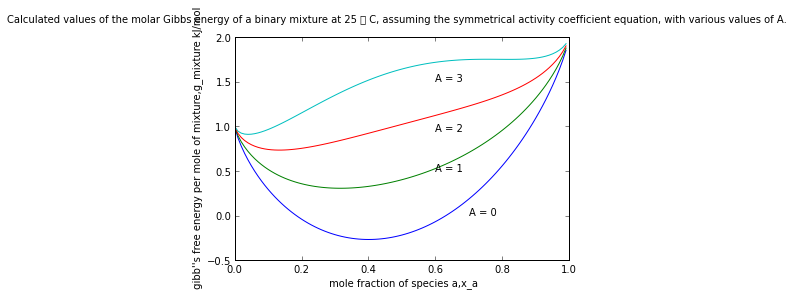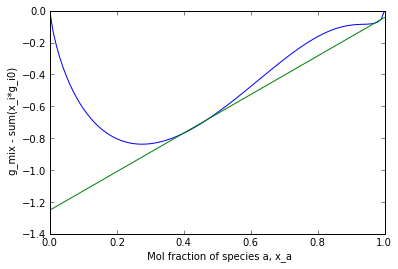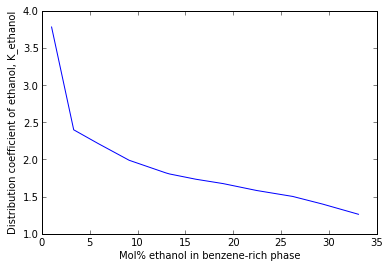# Chapter 11 : LIquid Liquid Liquid Solid And Gas Solid Equilibrium¶

### Example 11.1 Page: 272¶

In :
import math

V_water = 1.         #[L] volume of the water
Temp = 25.           #[C]
d_benzene = 0.88     #[g/ml] density of the benzene
M_benzene = 78.      #[g/mol] molecular weight of the benzene
M_water = 18.        #[g/mol]

n_1drop = 1./20*(d_benzene/M_benzene)       #[mol/drop]

n_water = 1000./M_water     #[mol]
s_benzene = 0.000405
n_benzene_saturate = s_benzene*n_water          #[mol]
N_benzene = n_benzene_saturate/n_1drop          #[drops]

print "The total number of the drops of the benznene required to saturate the water is %0.0f drops"%(N_benzene)

The total number of the drops of the benznene required to saturate the water is 40 drops


### Example 11.2 Page: 273¶

In :
import math

m_benzene = 1000.           #[lbm]
M_benzene = 78.             #[lbm/lbmol]
n_benzene = m_benzene/M_benzene        #[lbmol]
x_benzene = 0.000405

n_water = n_benzene/x_benzene       #[lbmol]
m_water = n_water*18                #[lbm]

print "The amount of the ground water that will make a saturated solution will be %e lbm"%(m_water)

The amount of the ground water that will make a saturated solution will be 5.698006e+05 lbm


### Example 11.3 Page: 277¶

In :
import math

Temp = 25.          #[C]
n_water = 3.75      #[mol]
n_ethanol = 2.5     #[mol]
n_benzene = 3.75    #[mol]

x_water = 0.375
x_ethanol = 0.250
x_benzene = 0.375

x_water_w = 64.9        #[%]
x_ethanol_w = 31.75     #[%]
x_benzene_w = 3.37      #[%]

x_water_b = 6.43        #[%]
x_ethanol_b = 18.94     #[%]
x_benzene_b = 74.62     #[%]

print "The composition of the two equilibrium phases i.e. water-reach phase and benzene reach phase is as "
print "\t\t\t\tWater-reach phase\t\tbenzene-reach phase"
print "Mol water\t\t\t%f\t\t\t\t%f"%(x_water_w,x_water_b)
print "Mol ethanol\t\t\t%f\t\t\t\t%f"%(x_ethanol_w,x_ethanol_b)
print "Mol benzene\t\t\t%f\t\t\t\t%f"%(x_benzene_w,x_benzene_b)

The composition of the two equilibrium phases i.e. water-reach phase and benzene reach phase is as
Water-reach phase		benzene-reach phase
Mol water			64.900000				6.430000
Mol ethanol			31.750000				18.940000
Mol benzene			3.370000				74.620000


### Example 11.4 Page: 282¶

In :
import math

Temp = 25.              #[C]
x_1in2 = 405.           #[ppm]
x_2in1 = 3000.          #[ppm]

x_water_w = (10**(6)-405.)/(10**(6))
x_benzene_w = 1-x_water_w

x_benzene_b =(10.**(6.)-3000.)/(10**(6))
x_water_b = 1-x_benzene_b

y_benzene = 1/(x_benzene_w)
y_water = 1/(x_water_b)

print " Activity coefficient of benzene in water is %f"%(y_benzene)
print " Activity coefficient of water in benzene is %f"%(y_water)

 Activity coefficient of benzene in water is 2469.135802
Activity coefficient of water in benzene is 333.333333


### Example 11.5 Page: 283¶

In :
import math
from numpy import *
from matplotlib.pyplot import *

%matplotlib inline

R = 8.314           #[J/(mol*K)] Universal gas consmath.tant
T = 298.15          #[K] Temperature
g_a_0 = 2.          #[kj/mol] Gibb's free energy of the pure species 'a'
g_b_0 = 1.          #[kj/mol] Gibb's free energy of the pure species 'b'

for a in range(4):
def f(x):
return  x*g_a_0 + (1-x)*g_b_0 + (R*T)/1000*(x*log(x) + (1-x)*log(1-x) + x*a*(1-x)**(2) + (1-x)*a*(x)**(2))

x=linspace(0.000001,0.990001,100)
a = f(x)
plot(x,a)

xlabel(" mole fraction of species a,x_a");
ylabel(" gibb''s free energy per mole of mixture,g_mixture kJ/mol");
annotate("A = 0",xy=(.7,0))
annotate("A = 1",xy=(.6,0.5))
annotate("A = 2",xy=(.6,0.95))
annotate("A = 3",xy=(.6,1.50))
suptitle("Calculated values of the molar Gibbs energy of a binary mixture at 25  C, assuming \
the symmetrical activity coefficient equation, with various values of A.")

show()
print " The plot is shown in the graphic window."

Populating the interactive namespace from numpy and matplotlib

WARNING: pylab import has clobbered these variables: ['f', 'draw_if_interactive']
%pylab --no-import-all prevents importing * from pylab and numpyThe plot is shown in the graphic window.


### Example 11.6 Page: 283¶

In :
import math
from matplotlib.pyplot import *
from numpy import *

T = 92 + 273.15         #[K] Temperature of the system
R = 8.314               #[m**(3)*Pa/(mol*K)] universal gas consmath.tant

A = 1.2739
B = 3.9771

def f(x_a):
return  (R*T/1000)*( x_a*log(x_a) + (1-x_a)*log(1-x_a) + \
x_a*(B**(2)*A*(1-x_a)**(2)/(A*x_a + B*(1-x_a))**(2)) + (1-x_a)* \
(A**(2)*B*x_a**(2)/(A*x_a + B*(1-x_a))**(2)) )

x_a = linspace(0.000001,0.99999,100)
a = f(x_a)
plot(x_a,a)

x = linspace(0.0,0.99999,100)
a = 1.2090312*x-1.251495764
plot(x,1.2090312*x-1.251495764)
xlabel(" Mol fraction of species a, x_a");
ylabel(" g_mix - sum(x_i*g_i0)");

show()

print "Thus based on the several assumptions that the Van Laar equation is an accurate representation of LLE"
print "we would conclude that at 92 deg C water-n-butanol does form two liquid phases."Thus based on the several assumptions that the Van Laar equation is an accurate representation of LLE
we would conclude that at 92 deg C water-n-butanol does form two liquid phases.


### Example 11.7 Page: 286¶

In :
import math

R = 8.314           #[J/(ml*K)]

h_mix = -2967.7*R/1000          #[kJ/mol] Heat of mixing of water-in-benzene

h_mix_1 = 522.9*R/1000          #[kJ/mol] Heat of mixing of benzene-in-water

print " Heat of mixing of water-in-benzene is given as %f kJ/mol"%(h_mix)
print " Heat of mixing of benzene-in-water is given as    %f kJ/mol"%(h_mix_1)

 Heat of mixing of water-in-benzene is given as -24.673458 kJ/mol
Heat of mixing of benzene-in-water is given as    4.347391 kJ/mol


### Example 11.8 Page: 287¶

In :
import math

T_i = 50.           #[F] Initial temperature of the system
T_f = 20.           #[F] Final temperature of the system
M_gas = 115.        #[g/mol] Molecular weight of gasoline at room temperature
M_water = 18.       #[g/mol] Molecular weight of water at the room temperaature
d = 720.            #[g/L] density of gasoline at the room temperature

s_50 = 0.00026      #[mol fraction]

s_20 = 0.0001       #[mol fraction]

s_rej = s_50 - s_20     # mol of water per mole of gasoline

w = (s_rej*d*M_water)/M_gas     #[g water/L gasoline]

print " The amount of water that will come out of the solution in the gasoline will be %f g water/L gasoline"%(w)
print " At 20 deg F we would expect this water to become solid ice, forming a piece large enough to plug the fuel line of a parked auto."

 The amount of water that will come out of the solution in the gasoline will be 0.018031 g water/L gasoline
At 20 deg F we would expect this water to become solid ice, forming a piece large enough to plug the fuel line of a parked auto.


### Example 11.9 Page: 290¶

In :
from numpy import *
from matplotlib.pyplot import *
import math

Temp = 25.      #[C]
x_water = 5.    #[mo]
x_benzene = 0.1 #[mol]

x_ethanol_water_rich = 3.817        #[%]
x_ethanol_benzene_rich = 1.010      #[%]

K = x_ethanol_water_rich/x_ethanol_benzene_rich

X = array([3.817,7.968,12.977,18.134,23.540,24.069,27.892,31.725,35.510,39.382,41.062,41.771])
Y = array([1.010,3.323,5.860,9.121,12.939,13.340,16.090,18.943,22.444,26.216,29.341,33.093])
Z = X/Y

plot(Y,Z)
xlabel("Mol% ethanol in benzene-rich phase ");
ylabel("Distribution coefficient of ethanol, K_ethanol");
show()
K_1 = 4

m_water_rich = 100*K_1/(K_1+1)
m_benzene_rich = 100/(K_1+1)

print " Ethanol''s 0.1 mol distributed in the water rich phase will be   %f mol%% of the total mol"%(m_water_rich)
print " Ethanol''s 0.1 mol distributed in the benzene rich phase will be %f mol%% of the total mol"%(m_benzene_rich)Ethanol''s 0.1 mol distributed in the water rich phase will be   80.000000 mol% of the total mol
Ethanol''s 0.1 mol distributed in the benzene rich phase will be 20.000000 mol% of the total mol


### Example 11.10 Page: 293¶

In :
import math

Temp = 20.          #[C]
s = 36.0            #[g per 100 g of water]
M_NaCl = 58.5       #[g/mol] molecular weight of NaCl
M_water = 18.       #[g/mol] molecular weight of water

w = s/(s+100)
w_percent = w*100       #[wt %]

x = (s/M_NaCl)/((s/M_NaCl)+(100/M_water))
x_percent = x*100       #[mol %]

print " Weight fraction of the NaCl in the saturated solution is %0.1f wt %%"%(w_percent)
print " Mol fraction of the NaCl in the saturated solution is    %0.0f mol %%"%(x_percent)

 Weight fraction of the NaCl in the saturated solution is 26.5 wt %
Mol fraction of the NaCl in the saturated solution is    10 mol %


### Example 11.11 Page: 293¶

In :
import math

T_inlet = 68.           #[F]
T_outlet = 110.         #[F]

s_inlet_carbonate = 60.         #[ppm]
s_inlet_sulphate = 2020.        #[ppm]

s_outlet_carbonate = 30.        #[ppm]
s_outlet_sulphate = 2000.       #[ppm]
# This is close enough to the solubility of the gypsum at 68F
# so we conclude that we would not expect either form of CaSO4 to prdcipitate

w = s_inlet_carbonate - s_outlet_carbonate         #[ppm]

print  " Total amount of the solid left behind in the heater will be        %0.1f ppm"%(w)

# Now if a typical houshold water heater heats 100 gallons/per day , we would expect to deposite
w_per_day = w*10**(-6)*100*8.33                    #[lb/day]
print  " Total amount of the solid left behind in the heater per day will be %.3f lb/day"%(w_per_day)

 Total amount of the solid left behind in the heater will be        30.0 ppm
Total amount of the solid left behind in the heater per day will be 0.025 lb/day


### Example 11.12 Page: 298¶

In :
import math

x_2 = 0.1
x_ideal_benzene = 0.114
x_ideal_CCl4 = 0.152

y_i_1 = x_ideal_benzene/x_2# corresponding to practically ideal solution

y_i_2 = x_ideal_CCl4/x_2# corresponding to mild type II behavior

print " Activity coefficient in benzene corresponding to practically ideal solution is %0.2f"%(y_i_1)
print " Activity coefficient in CCl4 corresponding to mild type II behavior         is %0.2f"%(y_i_2)

 Activity coefficient in benzene corresponding to practically ideal solution is 1.14
Activity coefficient in CCl4 corresponding to mild type II behavior         is 1.52


### Example 11.13 Page: 299¶

In :
import math

T = 273.15+20           #[K]

T_m = 273.15+800        #[K]
delta_h_fusion = 30219.         #[J/g]
R = 8.314               #[J/(mol*K)]

a = delta_h_fusion/(R*T)*(T_m/T-1)

x_NaCl_into_y_i_1 = 1/math.exp(a)

x_NaCl = 1/math.exp(a)*1

print " The solubility of the NaCl in water at 20 deg C is %e "%( x_NaCl)
print " But the experimental value is 0.1 so Similar to the results in book our results are very far wrong"

 The solubility of the NaCl in water at 20 deg C is 4.704794e-15
But the experimental value is 0.1 so Similar to the results in book our results are very far wrong


### Example 11.14 Page: 301¶

In :
import math

P = 1*14.7          #[psia]
T = 30.             #[F]
p_ice = 0.0808     #[psia]
x_water_in_ice = 1.00

y_water_vapour = x_water_in_ice*p_ice/P

print " Equilibrium concentration of water vapour in the air is %0.4f"%(y_water_vapour)

 Equilibrium concentration of water vapour in the air is 0.0055


### Example 11.15 Page: 302¶

In :
import math

T = 273.15+35           #[K]
p_d = 100.              #[atm]
R = 82.06               #[(cm**(3)*atm)/(mol*K)]

p_naphthalene = 0.00065         #[atm]

x_naphthalene = 1.00
P = p_d

y_naphthalene = x_naphthalene*p_naphthalene/P
v = 132.                #[cm**(3)/mol]
p_c = 1.                #[atm]
f_d_by_f_c = math.exp(v/(R*T)*(p_d-p_c))
y_naphthalene = f_d_by_f_c*y_naphthalene

print "Estimated solubility of naphthalene in CO2 is %e"%(y_naphthalene)

Estimated solubility of naphthalene in CO2 is 1.089816e-05

In [ ]: